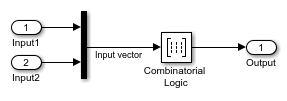Documentation

Two-Input AND Logic

This example builds a two-input AND function, which returns 1 when both input elements are 1, and 0 otherwise. To implement this function, specify the Truth table parameter value as [0; 0; 0; 1] The portion of the model that provides the inputs to and the output from the Combinatorial Logic block might look like this:The following table indicates the combination of inputs that generate each output. The input signal labeled Input corresponds to the column in the table labeled Input 1. Similarly, the input signal Input 2 corresponds to the column with the same name. The combination of these values determines the row of the Output column of the table that is passed as block output. For example, if the input vector is [1 0], the input references the third row:

(2^1*1 + 1) The output value is 0.